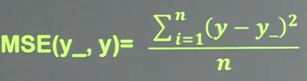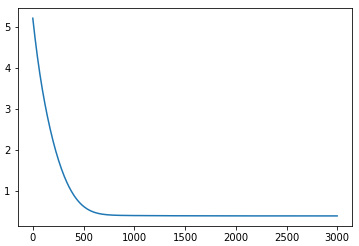# ​TensorFlow学习笔记（三）--反向传播

〇、瞎搞点数据

``````#设置常量，瞎编数据
#每次喂给神经网络几个数据
BATCH_SIZE = 8
#随机种子
seed = 23455
#基于seed产生随机数
rng = np.random.RandomState(seed)
X = rng.rand(32, 2)
Y = [[int(x0 + x1 < 1)] for (x0, x1) in X]
print("X:\n",X)
print("Y:\n",Y)``````

``````#占位符，以后存放训练集和对应的标签
x = tf.placeholder(tf.float32, shape=(None, 2))
y_= tf.placeholder(tf.float32, shape=(None, 1))``````

``````w1 = tf.Variable(tf.random_normal([2, 3], stddev=1, seed=1))
w2 = tf.Variable(tf.random_normal([3, 1], stddev=1, seed=1))``````

``````a = tf.matmul(x, w1)
y = tf.matmul(a, w2)``````

``loss = tf.reduce_mean(tf.square(y-y_))````````tf.train.GradientDescentOptimizer(LEARN_RATE).minimize(loss)
tf.train.MomentumOptimizer(LEARN_RATE, MOMENTUM).minimize(loss)

``train_step = tf.train.GradientDescentOptimizer(0.001).minimize(loss)``

``````#会话
with tf.Session() as sess:
init_op = tf.initialize_all_variables()
sess.run(init_op)
#开始训练
STEPS = 3000    #训练次数
for i in range(STEPS):
start = (i * BATCH_SIZE) % 32
end = start + BATCH_SIZE
sess.run(train_step, feed_dict={x:X[start:end], y_:Y[start:end]})
#每500次计算一次损失
if i % 500 == 0:
total_loss = sess.run(loss, feed_dict = {x:X, y_:Y})
print("Now %d steps, loss:%g" % (i, total_loss))``````MomentumOptimizer(0.001, 0.9)